### Understanding Self Attention and Positional Encoding Of The Transformer Architecture

Posted December 17, 2021 by Gowri Shankar  ‐  9 min read

The purpose of transformers architecture in deep learning AI models is to perform the transduction of one sequence of symbols into another. Transformers are nothing but clever utilization of matrix multiplication to infer the outcomes. They become popular due to their simplicity and a powerful replacement that answers the vanishing gradient issues of recurrent neural network models like LSTM(Long Short Term Memory) and GRU(Gated Recurrent Units). Often the most simple and admiring things that nature bestow upon us are the most mysterious things to comprehend when we dive deeper. Transformers fall into those categories of simple, elegant, trivial at face value but require superior intuitiveness for complete comprehension. Two components make transformers a SOTA architecture when they first appeared in 2017. First, The idea of self-attention, and Second, the Positional Encoding. Where attention mechanism is built quite clearly inspired by the human cognitive system and the positional encoding is purely a mathematical marvel.

Transformers are not new to us, we have studied them a few times in the past in the context of time series prediction(Temporal Fusion Transformers TFT). In this post, we are attempting to understand the two building blocks of transformers,

1. Self Attention and
2. Positional Encoding

The past writes of transformers can be referred to here,I thank the following folks who inspired me to write this, without their quality work this post, wouldn’t have been possible.

### Objective

The objective of this post is to understand the intuition behind Self Attention and the math behind Positional Encoding in transformer architecture. We have seen many blog posts and videos describing this concept by taking NLP as an example - I am attempting to take a different example where sequences are quite critical.

## Prologue

This post has two parts - One, understand self-attention with intuitive visualizations, and Two, Positional Encoding and the math behind it. We shall take the case of a journey from one city to another city with the various possible modes of transport to explain those 2 concepts.

Let us say we want to travel from the university town of Oxford, the UK to the picturesque city of Lyon, France. We depend on multiple modes of transportation to accomplish this journey because there is an English channel to cross. Let us portray this as a sequence,

### CASE 1 - First Order Sequence Model

$$Option \ 1 - By \ Ferry$$ $$\large Oxford, UK \rightarrow London, UK \rightarrow Dover \ Cliffs, UK \rightarrow Ferry \rightarrow Calais, FR \rightarrow Paris, FR \rightarrow Lyon, FR$$ $$Option \ 2 - By \ Euro \ Star$$ $$\large Oxford, UK \rightarrow London, UK \rightarrow Dover \ Cliffs, UK \rightarrow Euro \ Star \rightarrow Calais, FR \rightarrow Paris, FR \rightarrow Lyon, FR$$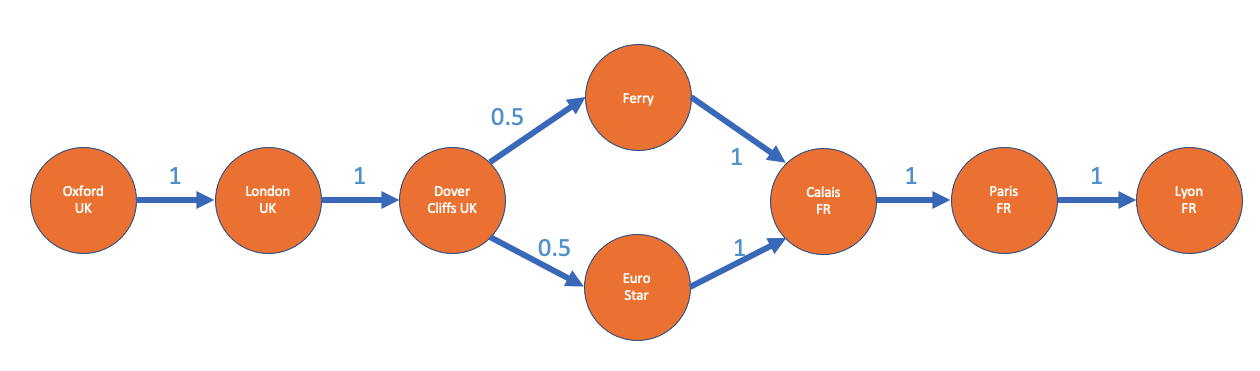$$Transition Matrix with Probability$$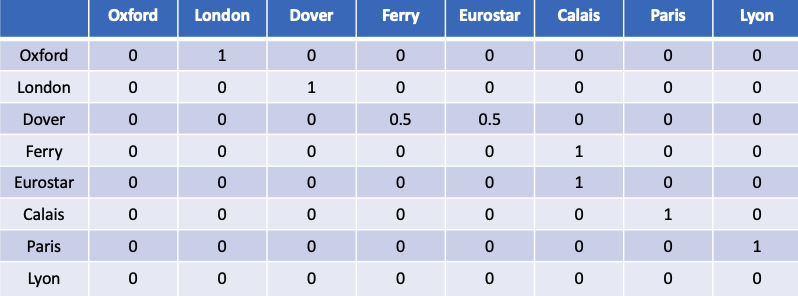### CASE 2 - Second Order Sequence Model

$$Option \ 1 - By \ Car \ and \ Train$$ $$\large Oxford, UK \rightarrow London, UK \rightarrow Euro \ Star + Car \rightarrow Paris, FR \rightarrow Car \rightarrow Lyon, FR$$ $$Option \ 2 - By \ Flight \ and \ TGV$$ $$\large Oxford, UK \rightarrow \ London, UK \rightarrow Flight \rightarrow Paris, FR \rightarrow TGV \rightarrow Lyon, FR$$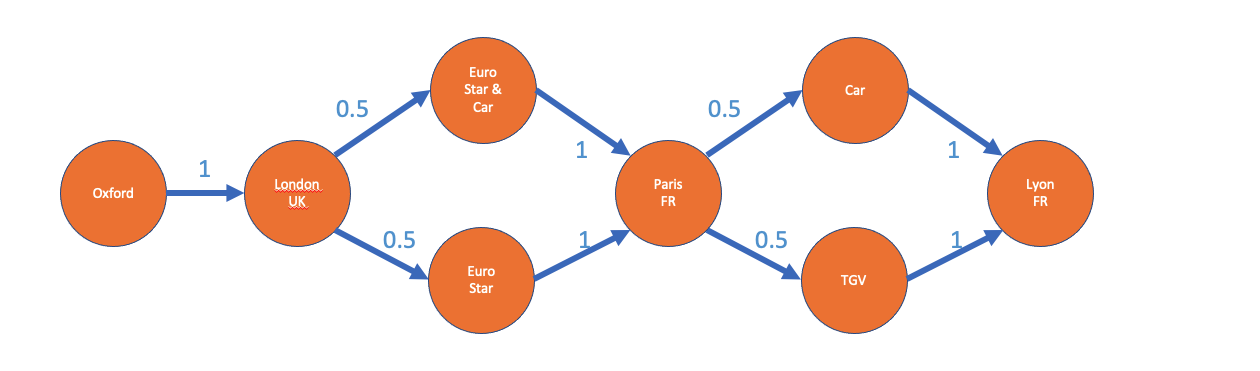$$Transition Matrix with Probability$$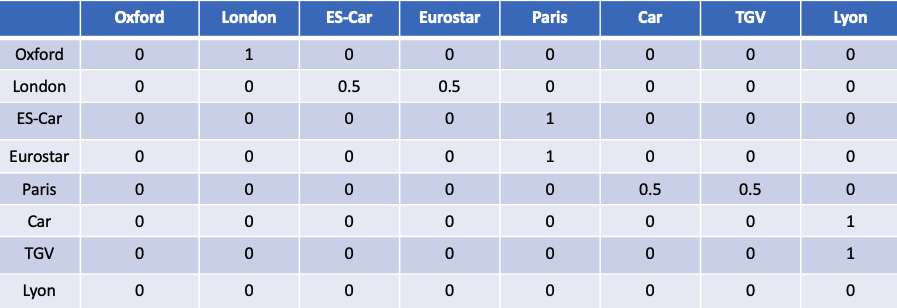The above scheme of travel can be called a Markov Chain - With probabilities mentioned.

### Self Attention

Case 1 discussed above is pretty straight forward but case 2 brings uncertainty in concluding. For the first order sequence model, we need to look at only one word to make the final decision. i.e. What is expected after reaching Dover Cliffs, taking Ferry and Eurostar has equal likelihood. On contrary, in the second-order sequence model - After reaching Paris, whether to travel by Car or TGV requires past information of whether we traveled in Eurostar with Car or Eurostar without Car. This idea of looking at all the information of the past for the current event is called Self Attention. Now the transition matrix for the second-order sequence model turns out to be

$$Second \ Order \ Transition \ Matrix$$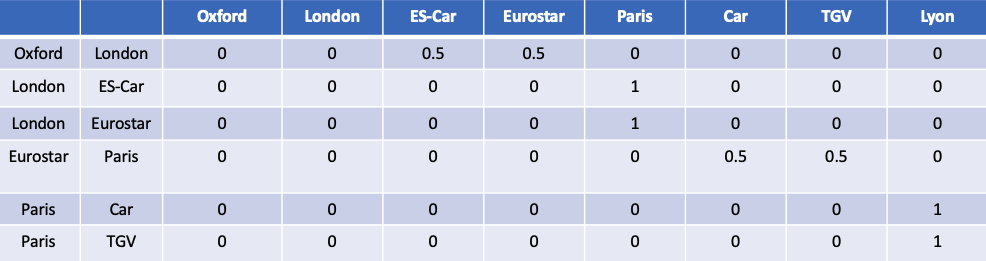If we closely observe the above matrix, there is quite a lot of irrelevant information there - Our only challenge is to figure out what is the mode of travel once we arrive at Paris. Then the transition matrix is deduced to the following one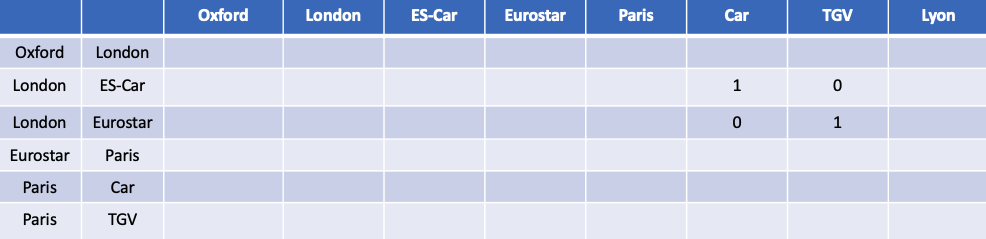If the mode of travel from London to Paris is Eurostar + Car then we are certain, the mode of travel from Paris to Lyon is Car otherwise, TGV. This is the intuition behind the Self Attention mechanism.

## Positional Encoding

Recurrent Neural Networks(RNNs) like LSTM and GRU successfully accommodate the order information of the sequences they deal with. However, a major problem occurs when the length of the sequence goes beyond certain dimensions - leading to a vanishing gradient problem. For convergence, we not only need a smooth gradient but also the gradient has to be remembered for long time steps. To make RNNs work for longer sequences, we need larger memory to process and the storage complexity and inherently the compute complexity shoots up during training time. This phenomenon makes RNNs not feasible for remembering the context in very long sequences.

On contrary, Transformers with their self and multi-headed attention being the backbone does not deal with sequence length but they consider the whole bunch of tokens to decide the next item in the sequence. i.e. With the attention mechanism alone, we cannot figure out the order of the words in the sentences, for example, where and whether an article or preposition or conjunction is required to make the sentence a meaningful one.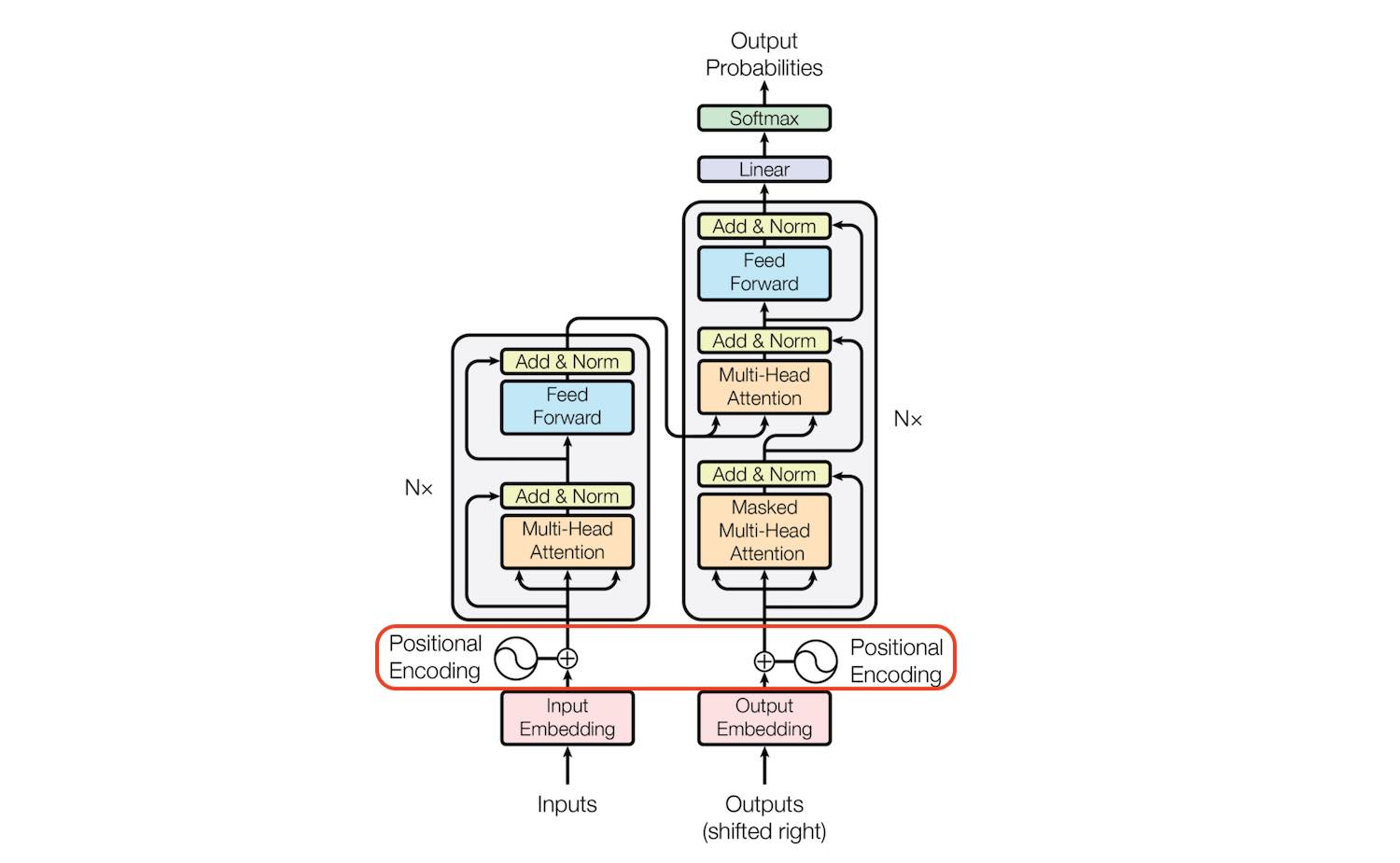Let us illustrate this with our toy example of traveling from Oxford to Lyon on what exactly Positional Encoding does. For simplicity, let us assume we are in the Eurostar with our car,

$$\large {London, Oxford}, Eursotor+Car \rightarrow Paris \Rightarrow {Oxford, London}, Eursotor+Car$$ Attention mechanism has no information about which one first, London or Oxford. Similarly, when we are in TGV $$\large {Paris, London, Eurostar, Oxford, }, TGV \rightarrow Lyon \Rightarrow {Oxford, London, Eurostar, Paris}, TGV$$

So the model gives only a bag full of items in the sequence but not their indices. Let us see the list of criteria mentioned by Amirhossein Kazemnejad,


It should output a unique encoding for each time-step
(word’s position in a sentence)

Distance between any two time-steps should be consistent
across sentences with different lengths.

Our model should generalize to longer sentences without
any efforts. Its values should be bounded.

It must be deterministic.




### Few Basics to Brush Up

Using embeddings we project the $N$ dimensional word representation into significantly lower dimension $d$, In the original Attention is all you need paper by Vaswani et al $d=512$. Where $N$ is the size of the vocabulary. So the first thing to learn in positional embedding is, It is yet another vector of length $d$ equal to the dimension $d$ of word embeddings. i.e $$d_{wordEmbedding} = d_{positionalEmbedding} \tag{1. Embedding Dimension}$$ Next aspects to learn are

• Positional embeddings are used to augment the input words with their positional information
• It is done by adding the positional embeddings to word embeddings - by choosing the dimension as same, a simple addition is quite possible $$\psi'(w_t) = \psi(w_t) + \vec{p_t} \tag{2. Positional Embeddings Augments Word Embeddings}$$

Where,

• $\psi'(w_t)$ is the actual embedding for every word $w_t$ in a sentence.
• $\vec{p_t}$ is the positional embedding

#### How $\vec{p_t}$ is calculated?

Calculating $\vec{p_t}$ turned out to be an outrageously clever scheme with absolute simplicity. To understand this, let us first recollect the way we represent numbers in binary.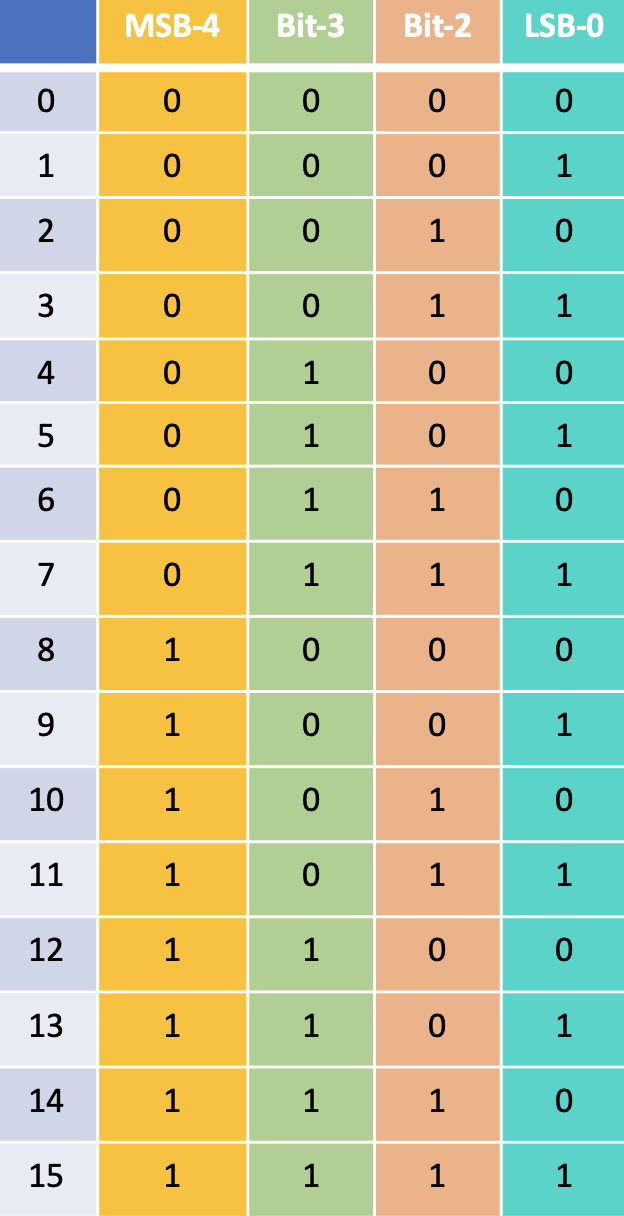The frequency at which each bit position transitioning from $0 \rightarrow 1$ and $1 \rightarrow 0$ is the inspiration for the positional encoding scheme. Representing binary values will result in space complexity and we are in the floating-point universe of continuous elements. The equivalent of alternating bits in the continuous world is Sinusoidal functions.

### Math Behind Positional Encoding

Conventional wisdom dictates us to use a single number to specify the location of an item, for example, indices in database tables. When we deal with continuous elements, a single number is not sufficient to represent and learn hence we use the $d$-dimenstional vector to specify a position as follows

$$\Large p_t^{(i)} = f(t)^{(i)} := \left{ \begin{array}{rc1} sin(w_t, t), & \mbox{if} & i =2k \ cos(w_t, t), & \mbox{if} & i = 2k +1 \end{array}\right. \tag{3. Positional Embedding}$$

Where,

• $\vec{p_t}$ is the desired encoded position in an input sequence
• $f(.)$ is the function that produces the positional vector $\vec{p_t}$ - Which is sinusoidals and $$w_k = \frac{1}{10000}^{2k/d}$$ It forms a geometric progression from $2\pi \rightarrow 10000.2\pi$ on the wavelengths

$\vec{p_t}$ is of the form containing vector pairs of sines and cosines for each frequency.

$$\Large \vec{p_t} = \left[\begin{array}{rc1} sin(w_1.t) \ cos(w_1.t) \ sin(w_2.t) \ cos(w_2.t) \ \vdots \ sin(w_n.t) \ cos(w_n.t) \ \end{array}\right]$$

### More Clarity from Brandon Rohrer

Brandon Rohrer’s explanation of transformers is one of the best in the web and it is quite recent. He had explained the positional encoding in a much more intuitive way, following are his words.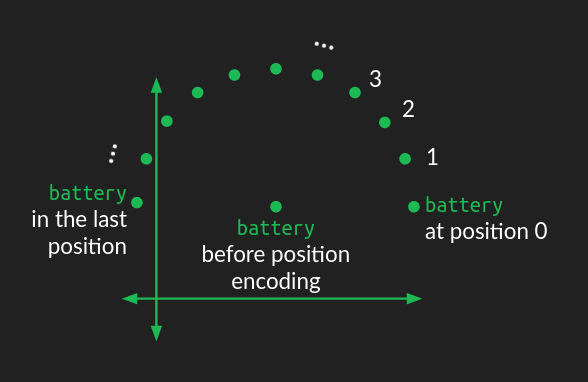The position of the word in the embedding space acts as the center
of a circle. A perturbation is added to it, depending on where it
falls in the order of the sequence of words. For each position, the
word is moved the same distance but at a different angle, resulting
in a circular pattern as you move through the sequence. Words that
are close to each other in the sequence have similar perturbations,
but words that are far apart are perturbed in different directions.

Since a circle is a two dimensional figure, representing a circular
wiggle requires modifying two dimensions of the embedding space. If
the embedding space consists of more than two dimensions (which it
almost always does), the circular wiggle is repeated in all the other
pairs of dimensions, but with different angular frequency, that is, it
sweeps out a different number of rotations in each case. In some
dimension pairs, the wiggle will sweep out many rotations of the circle.
In other pairs, it will only sweep out a small fraction of a rotation.
The combination of all these circular wiggles of different frequencies
gives a good representation of the absolute position of a word within
the sequence.

- Brandon Rohrer



## Epilogue

This is my $4^{th}$ post focusing on transformer and transformer architecture, I think learning new ideas from this one paper is never going to stop. The purpose of this post is to make a record of my learning and publish it for everyone’s review. This process often brought me awareness and identification of the areas I feel discomfort understanding. I am glad about this journey, pushing myself into uncharted territories always brings in the highest form of satisfaction and purpose to proceed forward. Hope you all enjoyed yet another post from my bag.

understanding-self-attention-and-positional-encoding-of-the-transformer-architecture